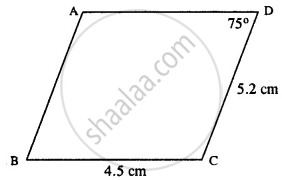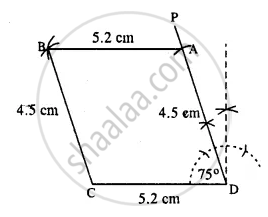# Construct a Parallelogram Abcd, If : Bc = 4.5 Cm, Cd = 5.2 Cm and ∠Adc = 75°. - Mathematics

Sum

Construct a parallelogram ABCD, if :

BC = 4.5 cm, CD = 5.2 cm and ∠ADC = 75°.

#### Solution

The rough figure is as follow :∵ opposite sides of ||gm are equal

∴ AD = BC = 4.5 cm.

∴ Actual construction is as follow:Steps :

1. Draw CD = 5.2 cm.
2. Draw ZCDP = 75°
3. Cut DA = 4.5 cm.
4. A drawn arc of radius 5.2 cm.
5. From C, draw an arc of radius 4.5 cm which meets the first arc at B.
6. Join AB and CB.
Thus ABCD is the required ||gm.
Concept: Construction of Parallelograms
Is there an error in this question or solution?

#### APPEARS IN

Selina Concise Mathematics Class 8 ICSE
Chapter 18 Constructions
Exercise 18 (D) | Q 2.2 | Page 211